Angle + comparing - examples

1. Gon functions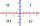Decide which of the numbers (values ​​of trigonometric functions) are positive and which are negative (or zero). Positive mark +1 and negative -1.
2. Angles and sides of the triangle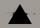Triangle ABC has a circumference of 26 cm. Lengths of the sides are as follows: a = 11.2 cm; b = 6.5 cm. Arrange the interior angles in order of its size. ?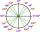What is greater angle? -0.7 radians or -0.7π radians?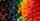Which of those angles are obtuse?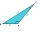Calculate the largest angle of the triangle whose side are 5.2cm, 3.6cm, and 2.1cm